#### 期刊菜单

Design and Implementation of a Software Phase-Locked Loop for Single-Phase PV Grid-Connected Inverter
DOI: 10.12677/OJCS.2019.82002, PDF , HTML, XML, 下载: 1,109  浏览: 4,348  国家自然科学基金支持

Abstract: Based on the closed-loop structure of traditional three-phase Phase-Locked loop, a software phase-locked loop (PLL) is proposed for single-phase PV grid-connected inverter in this paper. The operation principle of the presented phase-locked loop is analyzed and its mathematical model is deduced. Also, the software implementation method of the phase-locked loop is presented. Then, the influence resulted from the change of amplitude, frequency and phase on the grid voltage is simulated by PSIM software. The results show that the proposed software phase-locked loop can effectively and quickly realize the phase tracking at any time in the cycle, without waiting for the zero-crossing signal of the grid. The software phase-locked loop proposed in this paper is respectively applied to DSP TMS320F28035 and TMS320F2808 which belong to 500 W dual-channel single-phase PV grid-connected micro-inverter and 5 kW single-phase PV grid-connected inverter respectively. The results show that the proposed software phase-locked loop can achieve the voltage phase tracking and frequency locking well, thus verifying the proposed control method for single phase-locked loop.

1. 引言

2. 软件PLL原理

2.1. PLL原理

$\left\{\begin{array}{l}{v}_{d}={v}_{\alpha }\cdot \mathrm{cos}\theta +{v}_{\beta }\cdot \mathrm{sin}\theta \\ {v}_{q}={v}_{\beta }\cdot \mathrm{cos}\theta -{v}_{\alpha }\cdot \mathrm{sin}\theta \end{array}$ (1)

θ表示锁相环得到的相位角，vgrid表示电网电压。

$\left\{\begin{array}{l}{v}_{d}={v}_{\text{grid}}\cdot \mathrm{cos}\theta \\ {v}_{q}=-{v}_{\text{grid}}\cdot \mathrm{sin}\theta \end{array}$ (2)

$\left\{\begin{array}{l}{v}_{d}={V}_{\text{grid}}\cdot \mathrm{sin}\phi \cdot \mathrm{cos}\theta \\ {v}_{q}=-{V}_{\text{grid}}\cdot \mathrm{sin}\phi \cdot \mathrm{sin}\theta \end{array}$ (3)

$\left\{\begin{array}{l}{v}_{d}=\frac{{V}_{\text{grid}}}{2}\cdot \left[\mathrm{sin}\left(\phi -\theta \right)+\mathrm{sin}\left(\phi +\theta \right)\right]\\ {v}_{q}=\frac{{V}_{\text{grid}}}{2}\cdot \left[\mathrm{cos}\left(\phi +\theta \right)-\mathrm{cos}\left(\phi -\theta \right)\right]\end{array}$ (4)

 (5)(6)

$\frac{\text{d}{v}_{q}}{\text{d}t}=\frac{\text{d}{v}_{q}}{\text{d}\phi }\cdot \frac{\text{d}\phi }{\text{d}t}+\frac{\text{d}{v}_{q}}{\text{d}\theta }\cdot \frac{\text{d}\theta }{\text{d}t}$ (7)

$\frac{\text{d}{v}_{q}}{\text{d}t}=\frac{{V}_{\text{grid}}}{2}\cdot \left[-\mathrm{sin}\left(\phi +\theta \right)\right]\cdot \left(2\cdot {\omega }_{ff}\right)$ (8)

${\omega }_{err}=\frac{\text{d}{v}_{q}}{\text{d}t}\cdot \frac{1}{2{\omega }_{ff}}+{v}_{d}=\frac{{V}_{\text{grid}}}{2}\cdot \mathrm{sin}\left(\phi -\theta \right)$ (9)

2.2. 软件PLL的实现方法Figure 1. Control block diagram of phase-locked loop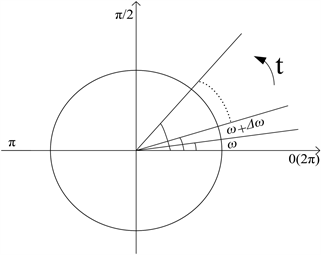Figure 2. Schematic diagram of phase superposition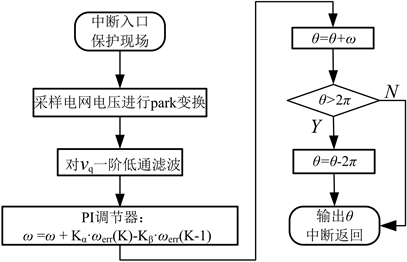Figure 3. Program flow diagram of PLL

vq对时间的微分在程序中是这样实现的：在s域，vq对时间的微分可以用 $s\cdot {v}_{q}$ 表示，对于 $s\cdot {v}_{q}$ 进行离散化可以得到如下式子：

 (10)

$\frac{1}{2{\omega }_{ff}}G\left(z\right)=\frac{{f}_{s}}{2{\omega }_{ff}}\cdot \left({v}_{q}-{v}_{q}\cdot {z}^{-1}\right)$ (11)

$\frac{{f}_{s}}{2{\omega }_{ff}}\left({v}_{q}\left(k\right)-{v}_{q}\left(k-1\right)\right)$ (12)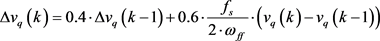(13)

3. PLL仿真

3.1. 软件PLL仿真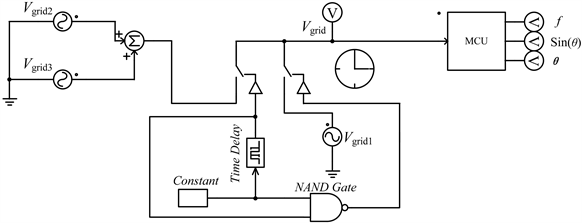Figure 4. Simulation circuit diagram of the phase-locked loop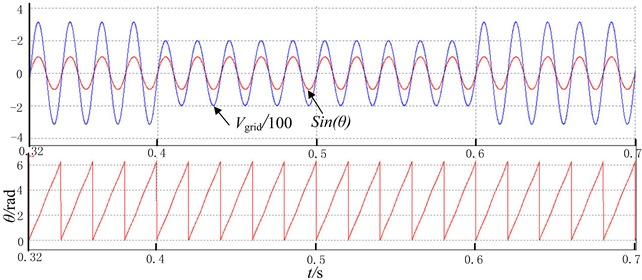Figure 5. Simulation diagram of voltage mutation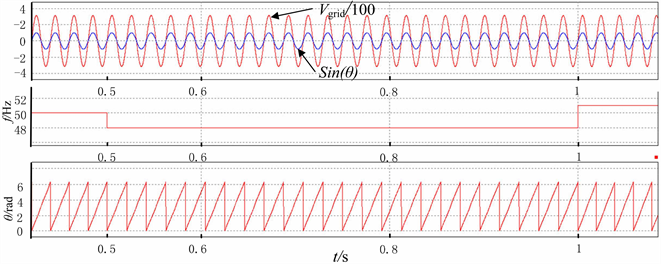Figure 6. Simulation diagram of frequency mutation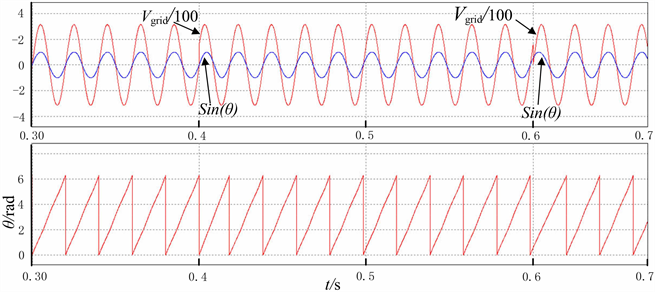Figure 7. Simulation diagram of phase mutation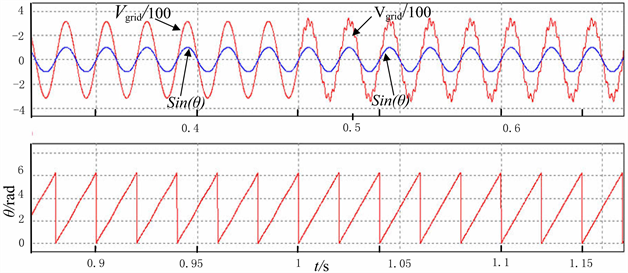Figure 8. Simulation diagram of phase locking with high order harmonics

3.2. 双通道微逆变器的仿真实现软件PLL算法

3.3. H6桥逆变器中的软件PLL算法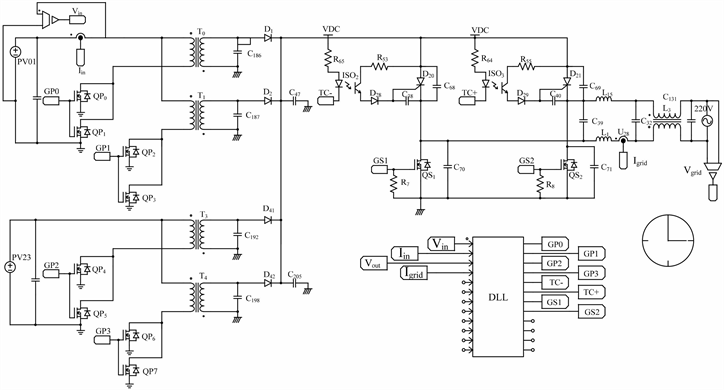Figure 9. Micro-inverter system simulation model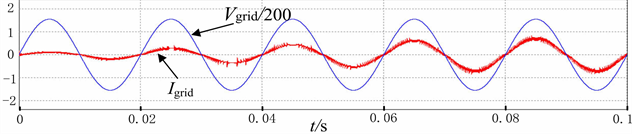Figure 10. Simulation waveform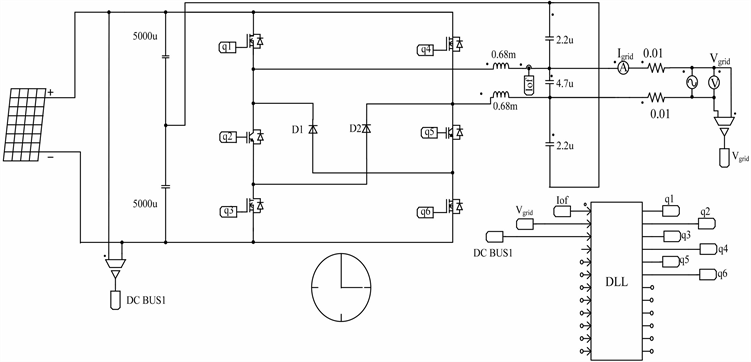Figure 11. Single-phase grid-connected inverter system simulation model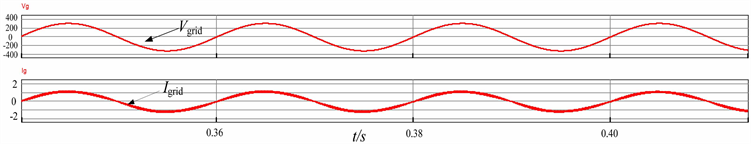Figure 12. Simulation waveform

4. 实验结果与分析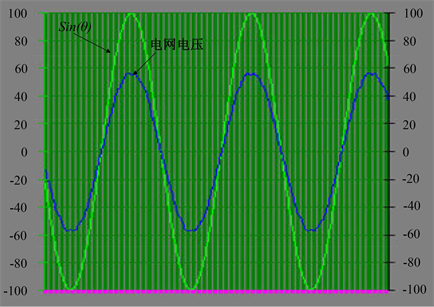Figure 13. Simulation diagram of phase mutation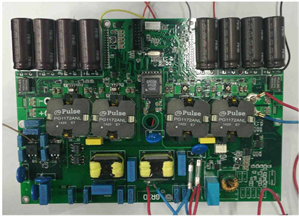Figure 14. Experimental prototype of the dual-channel micro-inverter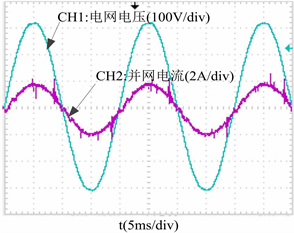Figure 15. Grid-connected experimental waveformsFigure 16. Experimental prototype of the single-phase grid-connected inverter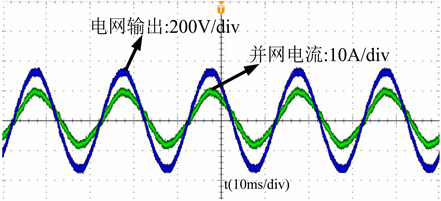Figure 17. Grid-connected experimental waveforms

  Zhang, C., Wang, X., Blaabjerg, F., Wang, W. and Liu, C. (2015) The Influence of Phase-Locked Loop on the Stability of Single-Phase Grid-Connected Inverter. IEEE Energy Conversion Congress and Exposition, Montreal, QC, 20-24 September 2015, 4737-4744. https://doi.org/10.1109/ecce.2015.7310329  宋杨呈祥. 应用于并网逆变器的锁相环关键技术[J]. 分布式能源, 2017, 2(3): 33-38.  郭子雷, 张海燕, 徐强, 秦臻. 新型锁相环技术及仿真分析[J]. 电测与仪表, 2015, 52(9): 82-86.  袁江伟, 吕征宇. 一种新型的单相并网系统锁相环[J]. 电力电子技术, 2011, 45(7): 81-82 + 100.  侯立健, 朱长青, 陈雅, 严雪飞. 基于二阶广义积分器的单相并网系统锁相技术的研究[J]. 电测与仪表, 2016, 53(13): 84-89.  赵贵龙, 曹玲玲, 祝龙记. 基于光伏并网逆变系统的改进锁相环设计[J]. 电力系统保护与控制, 2015, 43(2): 108-112.  严双喜, 张建文, 嵇伟, 俞良. 并网系统软件锁相环设计[J]. 电源技术, 2015, 39(10): 2277-2279.  刘翔, 张爱玲. 一种基于TMS320F2812的软件锁相环实现方法[J]. 电力电子技术, 2010, 44(8): 60-61.  唐轶, 谢永强, 王扬, 刘全景, 朱玉振. 基于解耦双同步坐标变换的三相锁相环研究[J]. 电测与仪表, 2017, 54(8): 14-20.  何攀, 席自强. 基于dq变换的锁相环设计与仿真[J]. 湖北工业大学学报, 2017, 32(5): 93-95.  Salman, A., Williams, A., Amjad, H., Bhatti, M.K.L. and Saad, M. (20105) Simplified Modeling of a PV Panel by Using PSIM and Its Comparison with Laboratory Test Results. 2015 IEEE Global Humanitarian Technology Conference, Seattle, WA, 8-11 October 2015, 360-364. https://doi.org/10.1109/ghtc.2015.7343997  Sahu, P.K., Shaw, P. and Maity, S. (2016) Modeling and Control of Grid-Connected DC/AC Converters for Single-Phase Micro-Inverter Application. 2015 Annual IEEE India Confer-ence (INDICON), New Delhi, India, 31 March 2016, 1-6. https://doi.org/10.1109/indicon.2015.7443537  李研达. 基于双派克变换的新型三相锁相环技术[J]. 沈阳工业大学学报, 2017, 39(3): 253-257.  徐亚伟. 并网逆变器中全软件锁相环的设计与实现[D]: [硕士学位论文]. 南京: 南京理工大学, 2014.  吴学敏, 吕鑫. 基于dq-PLL锁相环相序检测算法仿真研究[J]. 信息通信, 2016(7): 1-3.  瞿殿桂, 曹以龙. 基于双d-q旋转轴的三相电压锁相环分析及仿真[J]. 上海电力学院学报, 2017, 33(1): 49-54.  赵齐齐, 方宇, 张金银, 黎文, 王齐, 谢勇. 双反激型光伏并网微逆变器的建模及控制[J]. 电测与仪表, 2014, 51(10): 88-93.  张丽. 双通道交错反激型光伏并网微逆变器研究[D]: [硕士学位论文]. 扬州: 扬州大学, 2016.